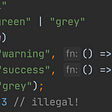# Rules of thumb for type constraints.

• Prefer manipulating data through interfaces rather than directly.
• If one typeclass can implement a second typeclass, the first typeclass is a subtype of the second.

At one point I needed to implement a simple stack structure in Haskell:

`import Data.Listtype Stack a = [a]push :: a -> Stack a -> Stack apush = (:)pop :: Stack a -> Maybe (a, Stack a)pop = uncons`

Nice and simple. Afterwards I needed to implement a stack somewhere else using a different data structure. In the interests of code reuse and simplicity, I decided to implement an interface:

`import Data.Listclass Stack s where    push :: a -> s a -> s a    pop :: s a -> Maybe (a, s a)instance Stack [] where    push = (:)    pop = uncons`

Which is only slightly longer than the original, with only one extra line of code. It’s usually going to be better to implement an interface and use that instead of using a data structure directly. We get the following advantages:

• Code reuse across all members of the typeclass.
• Fewer ways to write an incorrect program if we can only interact with a data structure through it’s interface.
• Less namespace pollution.
• Only marginally longer.

We can now implement a second instance (or more).

`data Deque a = Deque [a] [a]instance Stack Deque where    push y (Deque xs ys) = Deque xs (y: ys)    pop (Deque [] []) = Nothing    pop (Deque xs (y: ys)) = Just (y, Deque xs ys)    pop (Deque xs []) = pop (Deque [] (reverse xs))`

The implementation is unimportant, safe to say that it behaves like a stack.

I also wanted to fold across stack structures. For that we need to implement `Foldable` for all of the members of `Stack`. The minimum complete definition for `Foldable` is `foldr`. An example implementation of `foldr` could be as follows:

`foldrStack :: Stack s => (a -> b -> b) -> b -> s a -> bfoldrStack f z s = case pop s of    Nothing -> z    Just (x, s') ->  x `f` (foldrStack f z s')`

Which works for any type of `Stack`. My initial thought was to automatically implement `foldr` for every member of `Stack` automatically:

`-- Error.instance (Stack a) => Foldable a where    foldr = foldrStack`

Which doesn’t work. If it did work we could do the following:

`instance (Stack a) => Foldable a where    -- codeinstance (OtherClass a) => Foldable a where    -- code`

If we has something that instanced both `Stack` and `OtherClass`, the compiler would have no way of knowing which version of `Foldable` to use.

We know that every instance of `Stack` can also implement `Foldable` because we can write a default implementation for it. Therefore we should modify our definition of the `Stack` typeclass:

`-- make `Foldable` a prerequisite for `Stack`.class (Foldable s) => Stack s where    push :: a -> s a -> s a    pop :: s a -> Maybe (a, s a)instance Foldable Deque where    foldr = foldrStack-- Any other type of `Stack` can implement `Foldable` easily.instance Foldable OtherStack where    foldr = foldrStack`

The only disadvantage now is that we have to manually implement `Foldable` for every member of `Stack`. With our default implementation, this is usually pretty easy to do.

# Summary

• Try not to write functions for data structures directly, instead, try to access them through an interface. That way we can reuse functions across multiple instances.
• If you can write a default implementation for one typeclass using the functions from another typeclass, make it a type constraint. (e.g, we can make a default implementation for `Foldable`using the functions from `Stack`, so we make `Foldable` a type restriction).

--

--

--

The stories I write are a part of a learning journey through life, logic and programming. Share this journey with me.

Love podcasts or audiobooks? Learn on the go with our new app.## Andrew Pritchard

The stories I write are a part of a learning journey through life, logic and programming. Share this journey with me.

## Linting tools as a method of improving code quality in robust systems## Switch with a Functional and Generic Turn